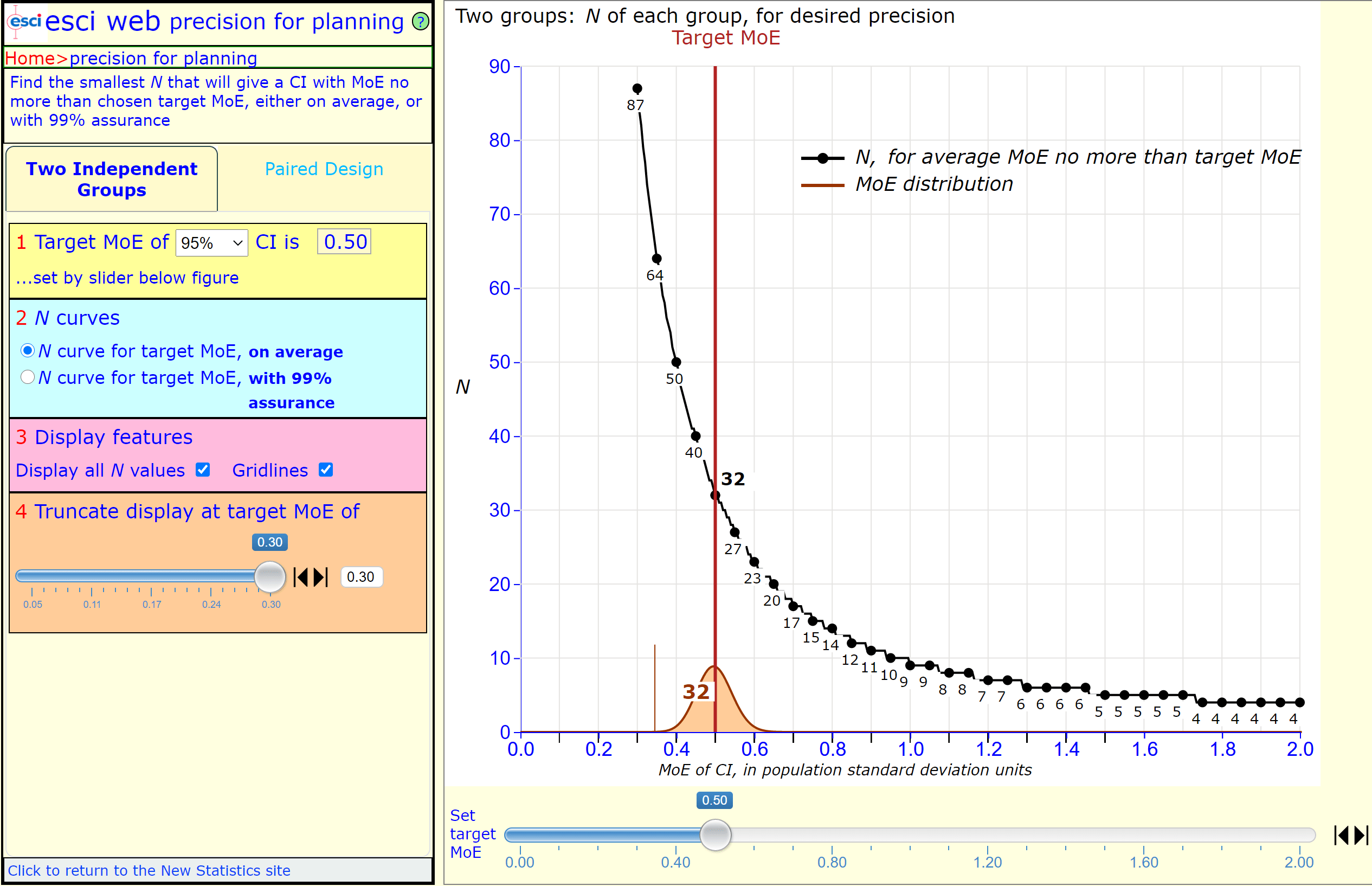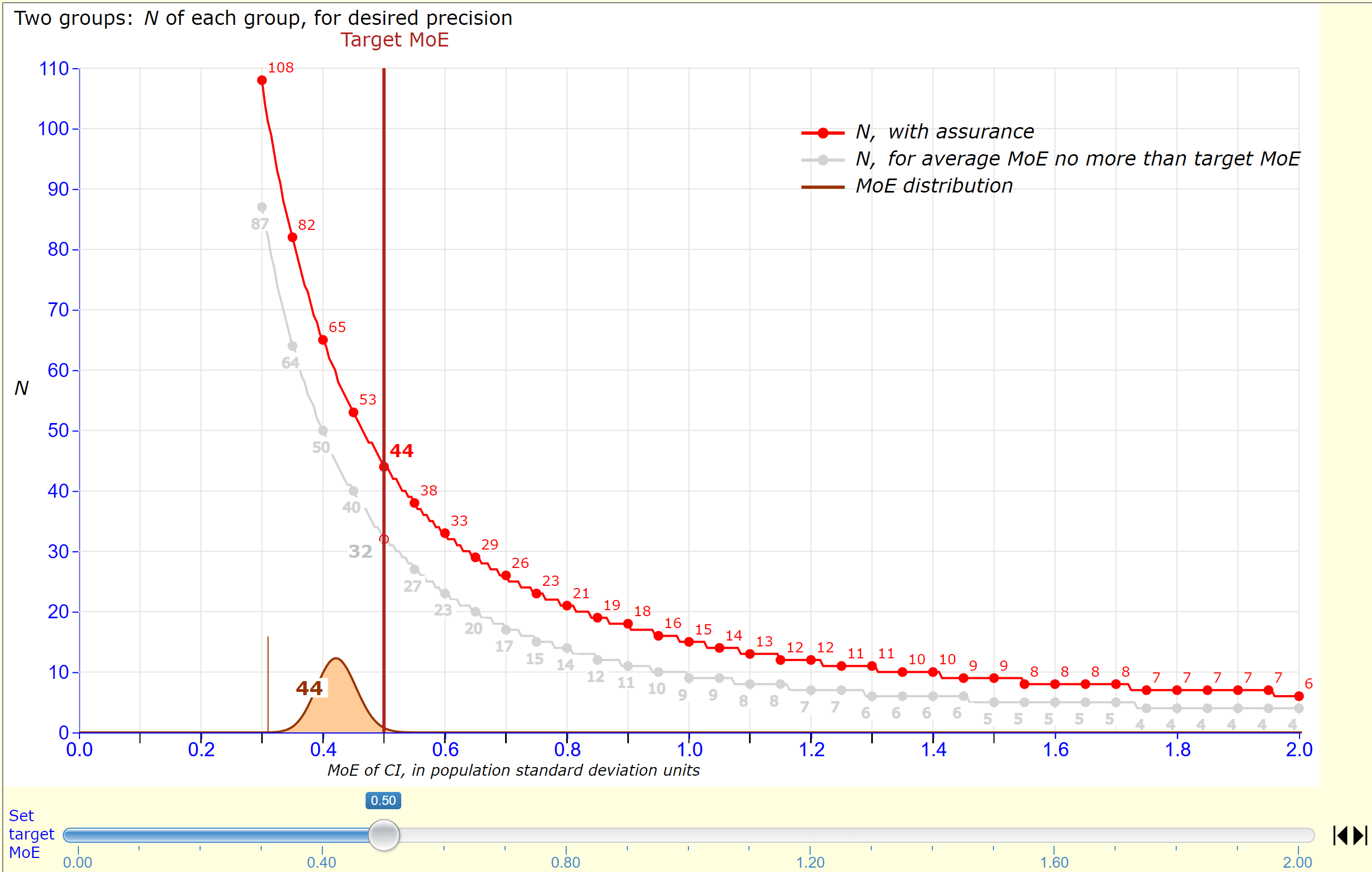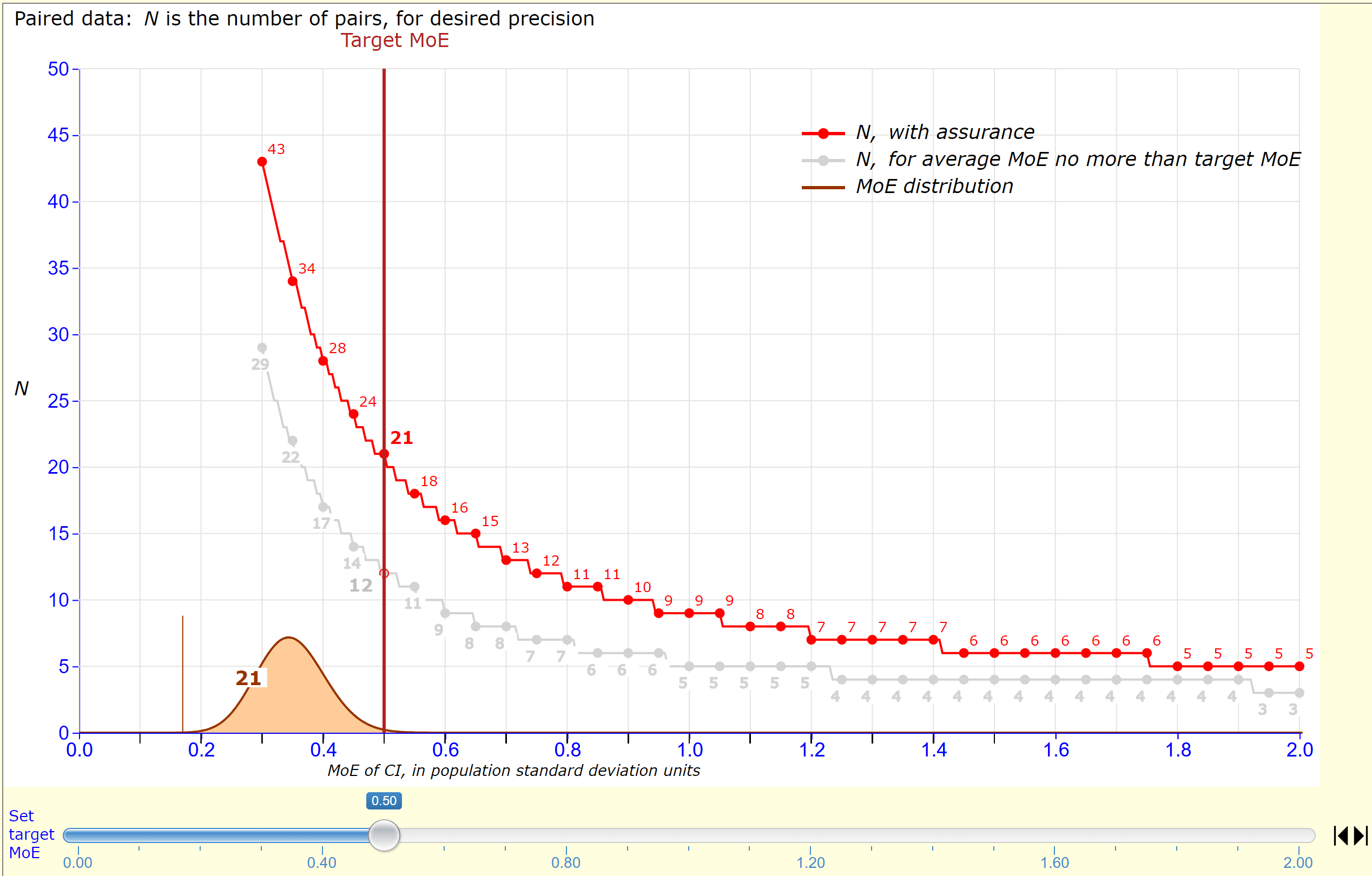# What N Will Give Me the Precision I Want? Gordon’s New Pictures Tell All

We’re delighted to release precision for planning (PfP), the sixth component of esci web. This completes esci web as currently planned. To access, click esci web and then the precision for planning button. Please let’s know how you like it, and give us your suggestions.

Precision for Planning tells us what N we need to achieve the precision we’d like. It’s a much better way to plan than the traditional use of statistical power, which works only within an NHST framework. Far better to adopt an estimation framework (the new statistics) and use PfP.

For an intro to PfP, see Chapter 10 in ITNS. For more detail, see Chapter 13 in UTNS.

### Two Independent Groups

For a two independent groups study, with two groups of size N, below is the PfP picture. Recall that MoE is the margin of error, which is half the length of a CI. I’ve set the slider at the bottom to target MoE = 0.50, meaning that I want to estimate the difference between the group means with a 95% CI having MoE of 0.50. In other words, each arm of the CI should be 0.50 in length.precision for planning from esci web. The large slider marks our selected target MoE of 0.50. Two groups each with N = 32 will, on average, give a 95% CI with MoE no more than 0.50. The lower curve is the distribution of MoE lengths when N = 32.

The lower axis is marked in units of population SD, which we can think of as units of Cohen’s d. The cursor marks a target MoE of 0.50 in those units.

The black curve shows how required N increases dramatically as we aim for smaller values of MoE–in other words, greater precision and a shorter CI. Use this curve to investigate how N trades with likely precision.

The small curve at the bottom shows how MoE varies for N = 32. It’s usually close to 0.50, but can be as short as 0.40 or long as 0.60, and occasionally even a little outside that range. Use the large slider to move the cursor and see the MoE distribution for other values of target MoE and N.

The figure gives us a handy benchmark, worth remembering: Any study with two independent groups of size 32 will estimate the difference between the group means with a 95% CI that has MoE of 0.50, on average.

### With 99% Assurance

The black curve can only give us N for MoE that’s sufficiently small on average. But we can do better. The red curve, below, tells us the N we need to achieve target MoE with assurance of 99%. This is the N that gives MoE smaller than target MoE on at least 99% of occasions. The grey curve reminds us of the ‘on average’ curve–the black curve in the figure above.precision for planning from esci web. The red curve shows N required with 99% assurance, meaning that MoE will be no more than target MoE on at least 99% of occasions. Two groups each with N = 44 will give MoE no more than 0.50 on at least 99% of occasions. The small lower curve is the distribution of MoE lengths when N = 44. Only the tiny right tail, area at most 1%, exceeds 0.50.

### The Paired Design

precision for planning supports PfP for what are probably the two most common designs: two independent groups, and the paired design. The paired design, with a single repeated measure (for example Pretest-Posttest) has the advantage, where it is possible and appropriate, of usually giving higher precision. The critical feature is the correlation in the population between the two measures, such as Pretest and Posttest. Higher correlation gives a shorter CI on the paired difference and therefore higher precision.

To use PfP we need to specify a value for ρ (Greek rho), the population correlation. Ideally, previous research gives us a reasonable estimate we can use; otherwise we might have to guess. For research with human participants, typical values are often around .6 to .9.

Here’s a PfP picture for the Paired Design, with ρ set to .70.precision for planning for the Paired Design, with ρ set to .70. Target MoE is 0.50. The grey curve tells us a single group of N = 12 will give MoE no more that 0.50, on average. The red curve shows that N = 21 will achieve that target MoE with assurance. The lower curve is the distribution of MoE lengths when N = 21.

The red curve shows us that a single group of N = 21 suffices for target MoE = 0.50 with assurance, when ρ = .70. Compare with two groups of N = 44 for the independent groups design. Great news!

However, as you might guess, N is highly sensitive to ρ. For ρ = .60 we need N = 25, but for ρ = .80 we need only N = 16 (or N = 9, on average).

It’s wonderful that precision for planning makes it easy to explore how N, target MoE, choice of design, and–for the Paired Design–ρ, all co-vary. Be fully informed before you choose a design and N!

### Full esci web

Go to esci web and see all six components as here: